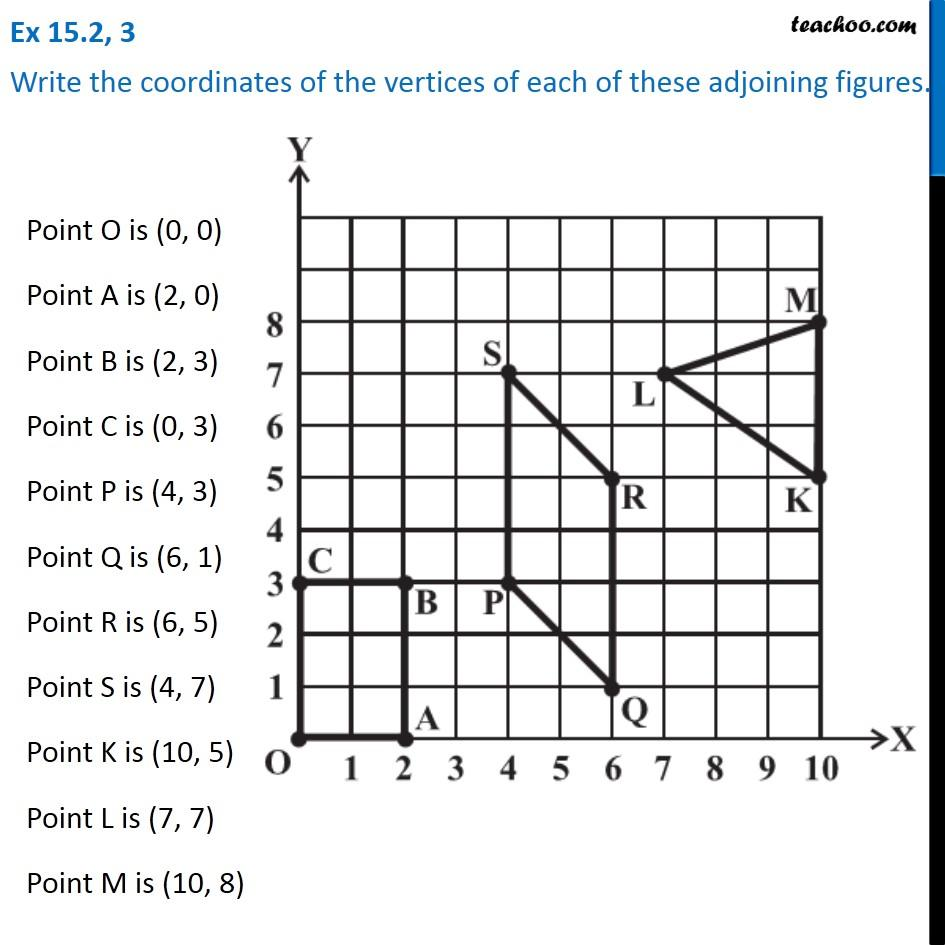Linear Graphs

Chapter 15 Class 8 Introduction to Graphs
Concept wiseIntroducing your new favourite teacher - Teachoo Black, at only ₹83 per month

### Transcript

Ex 15.2, 3 Write the coordinates of the vertices of each of these adjoining figures.Point O is (0, 0) Point A is (2, 0) Point B is (2, 3) Point C is (0, 3) Point P is (4, 3) Point Q is (6, 1) Point R is (6, 5) Point S is (4, 7) Point K is (10, 5) Point L is (7, 7) Point M is (10, 8)